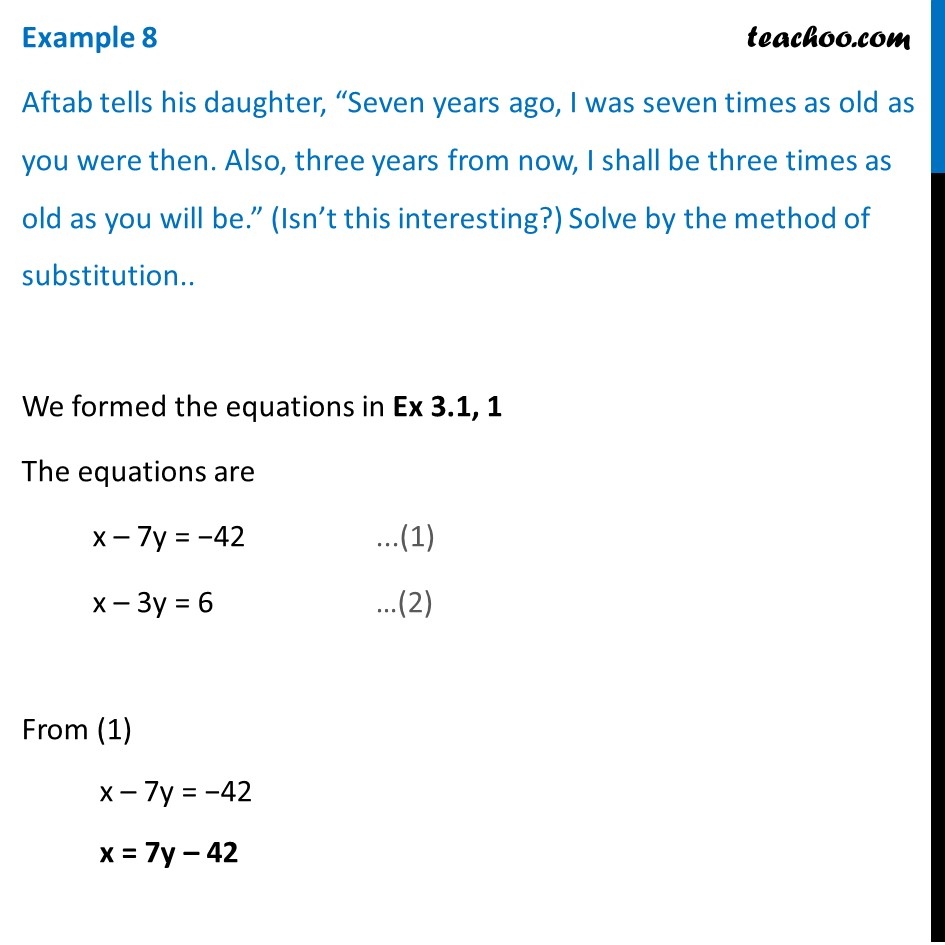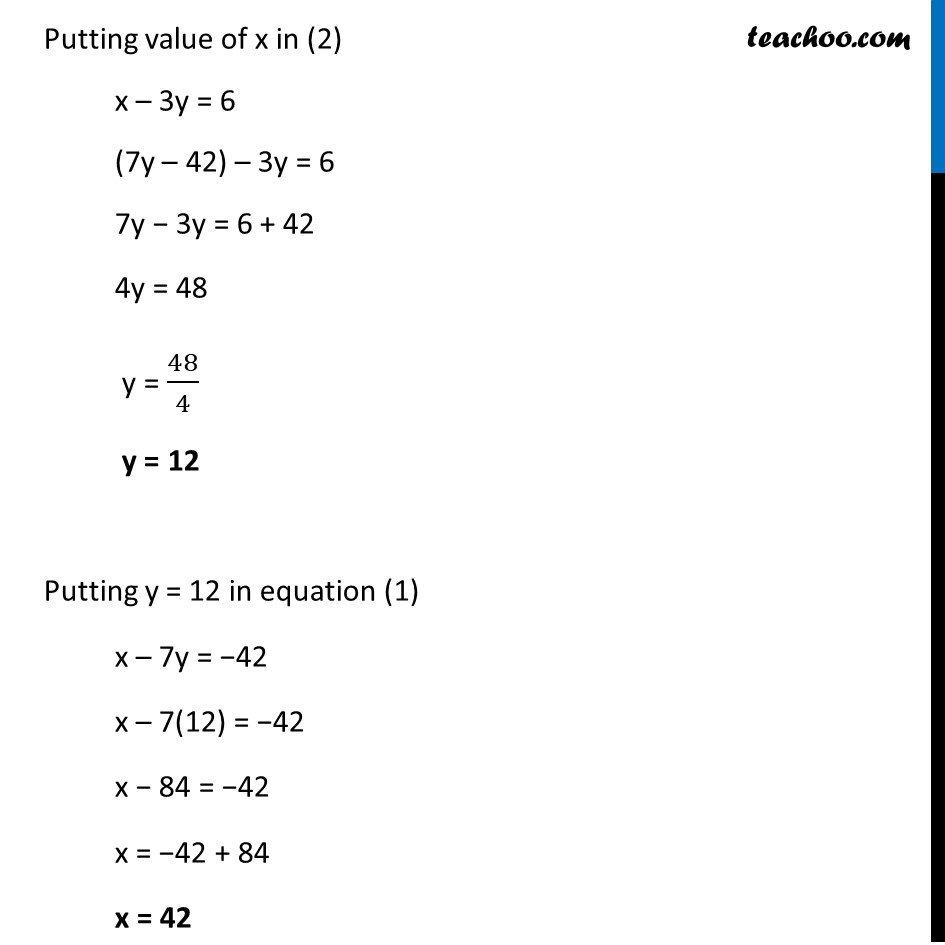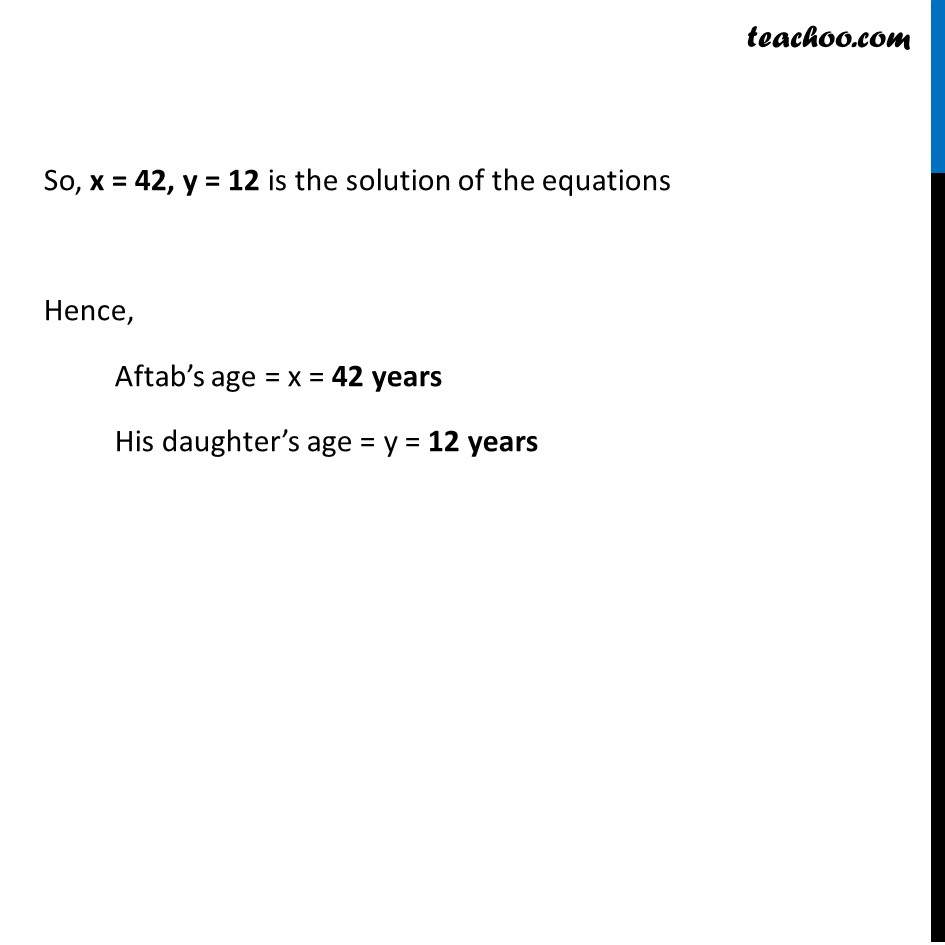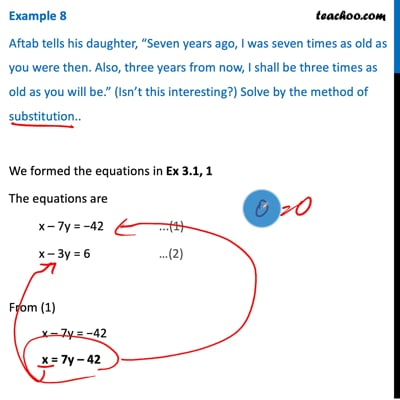Examples

Chapter 3 Class 10 Pair of Linear Equations in Two Variables
Serial order wiseThis video is only available for Teachoo black users

Maths Crash Course - Live lectures + all videos + Real time Doubt solving!

### Transcript

Example 8 Aftab tells his daughter, “Seven years ago, I was seven times as old as you were then. Also, three years from now, I shall be three times as old as you will be.” (Isn’t this interesting?) Solve by the method of substitution.. We formed the equations in Ex 3.1, 1 The equations are x – 7y = −42 ...(1) x – 3y = 6 …(2) From (1) x – 7y = −42 x = 7y – 42 Putting value of x in (2) x – 3y = 6 (7y – 42) – 3y = 6 7y − 3y = 6 + 42 4y = 48 y = 48/4 y = 12 Putting y = 12 in equation (1) x – 7y = −42 x – 7(12) = −42 x − 84 = −42 x = −42 + 84 x = 42 So, x = 42, y = 12 is the solution of the equations Hence, Aftab’s age = x = 42 years His daughter’s age = y = 12 years